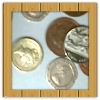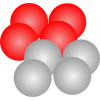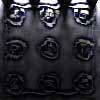#### You may also like### Win or Lose?

A gambler bets half the money in his pocket on the toss of a coin, winning an equal amount for a head and losing his money if the result is a tail. After 2n plays he has won exactly n times. Has he more money than he started with?### Fixing the Odds

You have two bags, four red balls and four white balls. You must put all the balls in the bags although you are allowed to have one bag empty. How should you distribute the balls between the two bags so as to make the probability of choosing a red ball as small as possible and what will the probability be in that case?### Scratch Cards

To win on a scratch card you have to uncover three numbers that add up to more than fifteen. What is the probability of winning a prize?

# In a Box

##### Age 14 to 16Challenge Level

You might like to draw your own tree diagrams for these solutions.Several of you suggested that having the same number of the same colour ribbons would make the game fair but this is not the case. Take, for example, one that many of you chose, which was four red and four blue ribbons. What happens?

\begin{eqnarray}\mbox{P (two the same colour)}&=& \mbox{P (Red and Red)}+\mbox {P (Blue and Blue)}\\ &=& \mbox{P(RR)}+\mbox{P(BB)}\\ &=&4/8 \times3/7 + 4/8 \times3/7 = 24/56 \end{eqnarray}

But this is less than a half so you have less chance of selecting two of the same colour than choosing two ribbons of different colours.

Laura and David of Cannock Chase High School showed that the game is not fair with 4 ribbons of one colour and two of the other
The game is not fair because:
$$2/6 \times1/5 = 2/30$$
$$4/6 \times3/5 =12/30$$

Probability that the two ribbons are the same is $14/30$ $(2/30 + 12/30)$.

The game is not fair as it is not an even chance; if it was even it would be $15/30$.

Nathan H. and Colby B. offered a solution to making the game fair:
There only need to be 4 ribbons (3 same, 1 different) therefore there can only be a 50/50 chance that one will win.

Working:

\begin{eqnarray}\mbox{P (Red and Red)} &=& \mbox{P (R)} \times \mbox{P (R)} \\ &=& 3/4 \times 2/3 \\ &=& 6/12 \; \mbox{OR}\; 1/2 \end{eqnarray}
\begin{eqnarray}\mbox{P (Red and Blue)} &=& \mbox{P (B)} \times \mbox{P (R)} \\ &=& 3/4 \times1/3 \\ &=& 3/12 \; \mbox{OR}\; 1/4 \end{eqnarray}
\begin{eqnarray}\mbox{P (Blue and Red)} &=& \mbox{P (R)} \times \mbox{P (B)} \\
&=& 1/4 \times3/3\\
&=& 3/12 \; \mbox{OR}\; 1/4 \end{eqnarray}
$$\text{P (Same)} = 1/2$$
$$\text{P (Different)} = 1/2$$

There is another way to even the game: Put 2 ribbons of one colour and 2 of another, and they each have to get the same colour (Red or Blue).

Amy of Kingwinford Secondary School suggested the following modification to the game to make it fair. Is she right?
There is more than one way to make a fair game - one way is:

The two players pick 1 ribbon, and say 1 player picks a red ribbon and the other picks a blue ribbon, then 1 point would get added on to you score, because you have both picked 2 different colours, but if they both picked the same colour then they would have points taken off their score.

There is another way of playing the game but differently, you could have 4 different coloured ribbons (red, blue, green and yellow) 2 of each colour then the players have to try and pick 2
ribbons of the same colour, if they do they win!

Andrei of School 205, Bucharest started looking for general solutions to a fair game and found some interesting patterns:

To solve the problem, I used combinations. The formula of combinations, i.e numbers of groups of k objects taken from n objects, where the order does not matter is:  $${n\choose{r}} = \frac{n!}{r!(n - r)!}$$ I calculated the number of pairs of ribbons of the same colour. There are two red ribbons and I take two: $${2\choose2}$$ There are four blue ribbons and I take two: $${4\choose2}$$ The total is: $${2\choose2} + {4\choose2}$$ In total, there are 6 ribbons, and I take 2. So, the total number of groups of 2 ribbons that could be formed from 6 is $${6\choose2}$$ The probability that the two ribbons are of the same colour (that Jo wins) is: $$\frac{{2\choose2} + {4\choose2}}{{6\choose2}} = \frac{1 + 6}{15} = \frac{7}{15}$$ Now, the probability that the two ribbons are of different colours (that Chris wins) is: $$1 - \frac{7}{15} = \frac{8}{15}$$ As the two probabilities are not equal, the game is not fair. Now, I try to find possibilities to make the game fair. I made a small program in which I introduced the two numbers, and it calculated the probability. The numbers I obtained formed an unexpected pattern. In the following table, the numbers in the first column represent the ribbons of the first colour, the numbers in the second column the ribbons of the second colour, while in the third column their difference is calculated:

 $1$ $3$ $3-1=2$ $3$ $6$ $6-3=3$ $6$ $10$ $10-6=4$ $10$ $15$ $15-10=5$ $15$ $21$ $21-15=6$ $21$ $28$ $28-21=7$

All the numbers are found on the third line of Pascal`s triangle, so they are triangular numbers.

Paris from IES Täby in Sweden sent in a full solution which shows algebraically the patterns that Andrei noticed.

Clickhere to see Paris' solution.